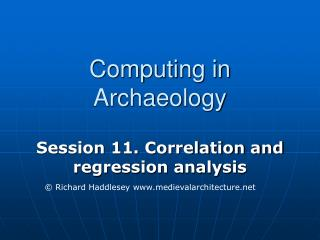# Computing in Archaeology - PowerPoint PPT PresentationDownload PresentationComputing in Archaeology

Computing in ArchaeologyDownload Presentation## Computing in Archaeology

- - - - - - - - - - - - - - - - - - - - - - - - - - - E N D - - - - - - - - - - - - - - - - - - - - - - - - - - -
##### Presentation Transcript

1. Computing in Archaeology Session 11. Correlation and regression analysis © Richard Haddlesey www.medievalarchitecture.net

2. Lecture aims • To introduce correlation and regression techniques

3. The scattergram • In correlation, we are always dealing with paired scores, and so values of the two variables taken together will be used to make a scattergram

4. example • Quantities of New Forrest pottery recovered from sites at varying distances from the kilns

5. Negative correlation Here we can see that the quantity of pottery decreases as distance from the source increases

6. Positive correlation Here we see that the taller a pot, the wider the rim

7. Curvilinear monotonic relation Again the further from source, the less quantity of artefacts

8. Arched relationship (non-monotonic) Here we see the first molar increases with age and is then worn down as the animal gets older

9. scattergram • This shows us that scattergrams are the most important means of studying relationships between two variables

10. REGRESSION • Regression differs from other techniques we have looked at so far in that it is concerned not just with whether or not a relationship exists, or the strength of that relationship, but with its nature • In regression analysis we use an independent variable to estimate (or predict) the values of a dependent variable

11. Regression equation y = f(x) • y = y axis (in this case the dependent • f = function (of x) • x = x axis

12. y = f(x) y = x y = 2x y = x2

13. General linear equations • y = a + bx • Where y is the dependent variable, x is the independent variable, and the coefficients a and b are constants, i.e. they are fixed for a given data

14. Therefore: • If x = 0 then the equation reduces to y = a, so a represents the point where the regression line crosses the y axis (the intercept) • The b constant defines the slope of gradient of the regression line • Thus for the pottery quantity in relation to distance from source, b represents the amount of decrease in pottery quantity from the source

15. y = a + bx

16. least-squares

17. least-squares

18. least-squares

19. least-squares

20. y = a + bx

21. y = a + bx

22. y = 102.64 – 1.8x

23. CORRELATION

24. CORRELATION 1 correlation coefficient

25. CORRELATION 1 correlation coefficient 2 significance

26. CORRELATION • 1 correlation coefficient • r • 2 significance

27. CORRELATION • 1 correlation coefficient • r • -1 to +1 • 2 significance

28. Levels of measurement: • nominal – in name only • ordinal – forming a sequence • interval – a sequence with fixed distances • ratio – fixed distances with a datum point

29. Levels of measurement: • nominal • ordinal • interval • ratio

30. Levels of measurement: • nominal • ordinal • interval Product-Moment • Correlation Coefficient • ratio

31. Levels of measurement: • nominal • ordinal Spearman’s Rank • Correlation Coefficient • interval • ratio

32. The Product-Moment Correlation Coefficient

33. sample – 20 bronze spearheads length (cm) width (cm) n=20

34. r = nΣxy – (Σx)(Σy) g √[nΣx2 – (Σx)2] [nΣy2 – (Σy)2] length (cm) width (cm) n=20

35. r = nΣxy – (Σx)(Σy) g √[nΣx2 – (Σx)2] [nΣy2 – (Σy)2] n=20

36. r = nΣxy – (Σx)(Σy) g √[nΣx2 – (Σx)2] [nΣy2 – (Σy)2] n=20

37. r = nΣxy – (Σx)(Σy) g= +0.67 √[nΣx2 – (Σx)2] [nΣy2 – (Σy)2] n=20

38. Test of product moment correlation coefficient

39. Test of product moment correlation coefficient H0 : true correlation coefficient = 0

40. Test of product moment correlation coefficient H0 : true correlation coefficient = 0 H1 : true correlation coefficient ≠ 0

41. Test of product moment correlation coefficient H0 : true correlation coefficient = 0 H1 : true correlation coefficient ≠ 0 Assumptions: both variables approximately random

42. Test of product moment correlation coefficient H0 : true correlation coefficient = 0 H1 : true correlation coefficient ≠ 0 Assumptions: both variables approximately random Sample statistics needed: n and r